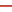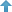# Change Signature in JavaScript

## Introduction

In JavaScript, you can use the Change Signature refactoring to:

• Change the function name.
• Add new parameters and remove the existing ones. Note that you can also add a parameter using a dedicated Extract Parameter refactoring.
• Reorder parameters.
• Change parameter names.
• Propagate new parameters through the method call hierarchy.

## Examples

The following table shows 4 different ways of performing the same Change Signature refactoring.

In all the cases, the function `result()` is renamed to `generate_result()` and a new parameter `input` is added to this function.

The examples show how the function call, the calling function (`show_result()`) and other code fragments may be affected depending on the refactoring settings.

BeforeAfter
```// This is the function whose signature will be changed: function\$#160;result() { // some code here } function show_result() { // Here is the function call: alert('Result: ' + result()); } // Now we'll rename the function and // add one (required) parameter.```
```// The function has been renamed to generate_result. // The new parameter input has been added. function generate_result(input) { // some code here } function show_result() { // The function call changed accordingly: alert('Result: ' + generate_result(100)); } // When performing the refactoring, 100 was specified as // the parameter value.```
```// This is the function whose signature will be changed: function result() { // some code here } function show_result() { // Here is the function call: alert('Result: ' + result()); } // Now we'll rename the function and add one parameter. // This time, we'll specify that the parameter is optional.```
```// The function has been renamed to generate_result. // The new optional parameter input has been added. function generate_result(input) { input = input || 100; // some code here } function show_result() { // The function call changed accordingly: alert('Result: ' + generate_result(100)); } // When performing the refactoring, 100 was specified as // the parameter value.```
```// This is the function whose signature will be changed: function result() { // some code here } // This function will also change its signature: function show_result() { // Here is the function call: alert('Result: ' + result()); } // Now we'll rename the function and add one required // parameter. We'll also ask PyCharm to propagate // the new parameter through the calling function show_result() // to the function call.```
```// The function has been renamed to generate_result. // The new parameter input has been added. function generate_result(input) { // some code here } // Note the new function parameter: function show_result(input) { // The function call changed accordingly: alert('Result: ' + generate_result(input)); }```
```// This is the function whose signature will be changed: function result() { // some code here } // This function will also change its signature: function show_result() { // Here is the function call: alert('Result: ' + result()); } // Now we'll rename the function and add one optional // parameter. We'll also ask PyCharm to propagate // the new parameter through the calling function show_result() // to the function call.```
```// The function has been renamed to generate_result. // The new optional parameter input has been added. function generate_result(input) { input = input || 100; // some code here } // Note the new function parameter: function show_result(input) { input = input || 100; // The function call changed accordingly: alert('Result: ' + generate_result(input)); } // When performing the refactoring, 100 was specified as // the parameter value.```

## To change a function signature

1. In the editor, place the cursor within the name of the function whose signature you want to change.
2. Do one of the following:
• Press Ctrl+F6.
• Choose Refactor | Change Signature in the main menu.
• Select Refactor | Change Signature from the context menu.
3. In the Change Signature dialog, make the necessary changes to the function signature and specify which other, related changes are required.

You can:

• Change the function name. To do that, edit the text in the Name field.
• Manage the function parameters using the table of parameters and the buttons to the right of it:
• To add a new parameter, click(Alt+Insert) and specify the properties of the new parameter in the corresponding fields.

When adding parameters, you may want to propagate these parameters to the functions that call the current function.

• To remove a parameter, click any of the cells in the corresponding row and click(Alt+Delete).
• To reorder the parameters, use(Alt+Up) and(Alt+Down). For example, if you wanted to make a certain parameter the first in the list, you would click any of the cells in the row corresponding to that parameter, and then clickthe required number of times.
• To change the name of a parameter, make the necessary edits in the corresponding table cell.
• Propagate new function parameters (if any) along the hierarchy of the functions that call the current function.

(There may be the functions that call the function whose signature you are changing. These functions, in their turn, may be called by other functions, and so on. You can propagate the changes you are making to the parameters of the current function through the hierarchy of the calling functions and also specify which calling functions should be affected and which shouldn't.)

To propagate the new parameters:

1. Click.
2. In the left-hand pane of the Select Methods to Propagate New Parameters dialog, expand the necessary nodes and select the check boxes next to the functions you want the new parameters to be propagated to.

To help you select the necessary functions, the code for the calling function and the function being called is shown in the right-hand part of the dialog (in the Caller Method and Callee Method panes respectively).

As you switch between the functions in the left-hand pane, the code in the right-hand part changes accordingly.

3. Click OK.
4. To perform the refactoring right away, click Refactor.

To see the expected changes and make the necessary adjustments prior to actually performing the refactoring, click Preview.